Internal curves are curves that define a cross-sectional shape of a surface. Any number of internal curves can be added to a surface defined by boundaries, according to the following rules:
You cannot add a COS as an internal curve.
Internal curves cannot intersect consecutive (contignuous) boundaries in boundary surfaces, as shown in the following diagram.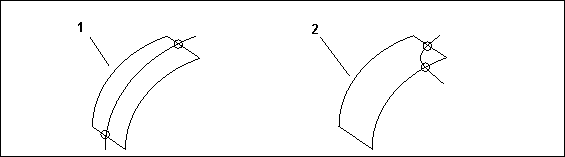1. Correct
2. Incorrect
Generally, internal curves must have soft points wherever they intersect the surface boundaries or other internal curves, as shown in the following diagram.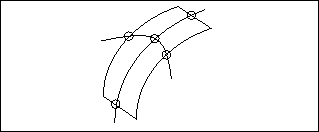If two internal curves cross the same borders in a boundary surface, they cannot intersect within the surface, as shown in the following diagram.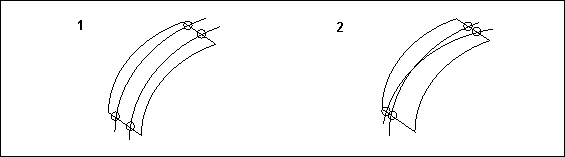1. Correct
2. Incorrect
The internal curve must intersect both borders of the surface, as shown in the following diagram.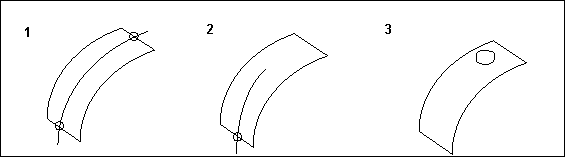1. Correct
2. Incorrect
3. Incorrect
An internal curve cannot intersect the surface boundary at more than two points, as shown in the following diagram.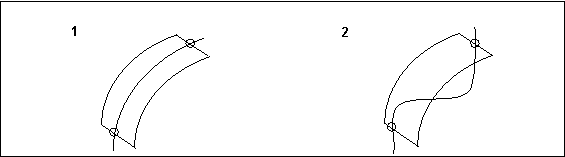1. Correct
2. Incorrect
Internal curves in triangular surfaces may or may not intersect the natural boundary.
An internal curve that intersects the natural boundary must pass through the degenerate vertex.
An internal curve that does not intersect the natural boundary must intersect the other two boundaries.